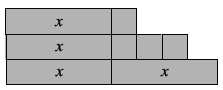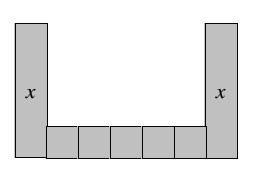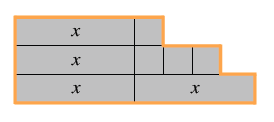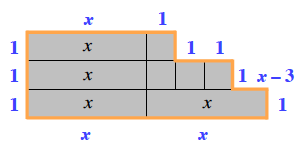### Home > MC2 > Chapter 4 > Lesson 4.2.3 > Problem4-60

4-60.

Find the perimeter and area of each algebra tile shape below. Be sure to combine like terms.

1.1.Remember, the perimeter is the sum of all the side lengths around the shape.

Labeling all the side lengths may prove to be more helpful for you.

Add up all these values to find the perimeter.

The area is the space within the blue lines.

Add up the values of the algebra tiles to find the area.

P $= 4x + 6$
A $= 4x + 4$See part (a).